python plotting tutorial - 1Weiran Huang Jan 13, 2018

Use matplotlib in Python to create wonderful graphs!

The following is the note I took when I was learning scientific computing with Python.

The book I use is Scientific computing with Python3 published by Packt and the contents in this article are mostly from this book. The copyright belongs to the author.

I used Jupyter notebook to generate this markdown file.

## Preface

Plotting in Python is done in the pyplot part in matplotlib module.
Here we import the module in this way:

from  matplotlib.pyplot import *


Since we are using Jupyter notebook, we start with the magic command “%matplotlib”

%matplotlib inline # in order to show plot in jupyter notebook


## Basic plotting

function: plot.
plot(x,y): a plot of y as a function of x (inputs are arrays/lists of equal length)

You can also use plot(y) as it is in fact short for plot(range(len(y)), y)

### example 1

The following is a plot drawing with this function. We plot the function $sin(x)$ for $x \in [-2\pi, 2\pi]$ using 200 sample points and set markers every fourth points.

from scipy import *
from matplotlib.pyplot import *

# plot sin(x) for some interval
x = linspace(-2*pi, 2*pi, 200)
plot(x, sin(x))

# plot markers for every 4th point
samples = x[::4]
plot(samples, sin(samples),"r*") # mark with red star-shape markers"

title("Function $sin(x)$ with some points marked")
grid()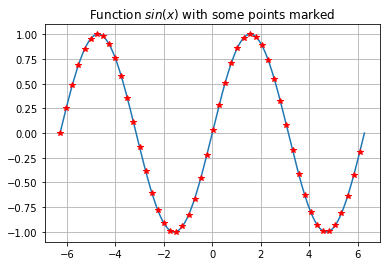To call a new clean window of plot, use function figure(). To switch from figures, using numbers, for example, figure(2). Without a number, python will create a new window.

### example 2

To add lebels, we use the function legend.
example:

# ---polyfit example---
x = range(5)
y = [1, 2, 1, 3, 5]
p2 = polyfit(x, y, 2)
p4 = polyfit(x, y, 4)

# plot the polynomials and points
xx = linspace(-1, 5, 200)
plot(xx, polyval(p2, xx), label = 'fitting polynomials of degree 2')
plot(xx, polyval(p4, xx), label = 'fitting polynomials of degree 4')
plot(x, y, '*')

# set the axis and legend
axis([-1, 5, 0, 6])
legend(loc = 'upper left', fontsize = 'small')

<matplotlib.legend.Legend at 0x19d0f9a75c0>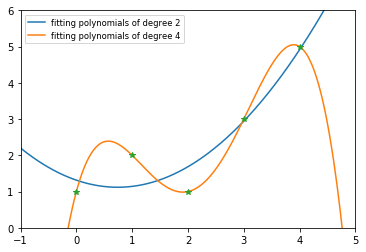### example 3

do scatter plots and logarithmic plots:

# create random 2D points
import numpy
x1 = 2 * numpy. random.standard_normal((2,100))
x2 = 0.8 * numpy.random.standard_normal((2,100)) + array([, ])
plot(x1, x1, '*')
plot(x2, x2, 'r*')
title('2D scatter plot')

Text(0.5,1,'2D scatter plot')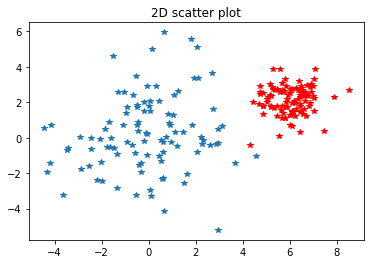the logarithmic plot using loglog:

# log both x and y axis
x = linspace(0,10,200)
linestyle = '-', linewidth = 3)

loglog(x,4*x**4, label = '4th degree polynomial',
linestyle = '-.', linewidth = 3)

loglog(x,5*exp(x), label = 'exponential function', linewidth = 3)

title('Logarithmic plots')
legend(loc = 'best')

<matplotlib.legend.Legend at 0x19d0fb20e80>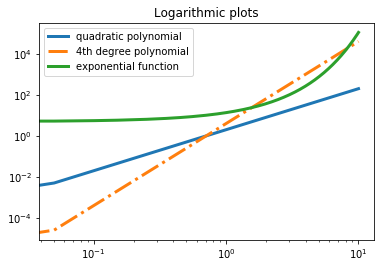## Formatting

The appearance of figures and plots can be styled and customized to look how you want them to look.
Some important variables are

1. linewidth, which controls the thickness of plot lines;
2. xlabel, ylabel, which set the axis labels;
3. color for plot colors;
4. transparent for transparency.

The following is an example with more keywords:

k = 0.2
x = [sin(2*n*k) for n in range(20)]
plot(x, color='green', linestyle='dashed', marker='o',
markerfacecolor='blue', markersize=12, linewidth=6)

[<matplotlib.lines.Line2D at 0x19d0fc342b0>]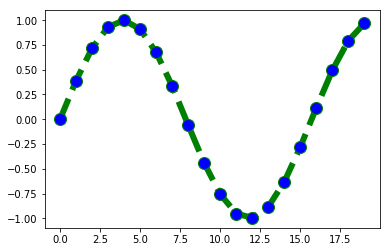You can use plot(...,'ro-'), or the more explicit syntax plot(..., marker='o', color='r', linestyle='-').

### hist function

To get histgram instead of normal plot, use the function hist.
example:

# random vector with normal distribution
sigma, mu = 2, 10
x = sigma * numpy.random.standard_normal(10000) + mu
hist(x, 50, normed = 1)
z = linspace(0, 20, 200)
plot(z, (1/sqrt(2*pi*sigma**2))*exp(-(z-mu)**2/(2*sigma**2)),'g')
# title with LaTeX formatting
title('Histogram with $\mu$ = {}, $\sigma$ = {}'.format(mu,sigma))

Text(0.5,1,'Histogram with $\\mu$ = 10, $\\sigma$ = 2')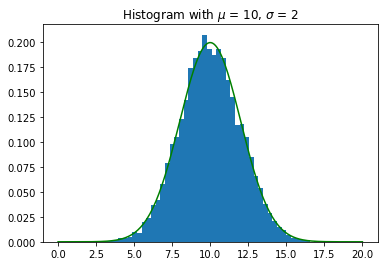• sometimes the string formatting will interfere with our LaTeX bracket. When this happens, we use double brackets in LaTeX, for example use x_1 instead of x_{1}.
• the text sometimes will also overlap with the escaping sequences. For example \tau will be interpreted as \t. In this case we should use raw string such as r'\tau'

### subplot function

To show several plot in the same window:
subplot(v, h, c)

v: the number of vertical plots.

h: the number of horizontal plots.

c: the index indicating which position to plot in (counted row-wise)

use subplot_adjust to add extra space to adjust the distance between different subplots.

def avg(x):
""" simple running average """
return (roll(x,1) + x + roll(x,-1)) / 3

# sine function with noise
x = linspace(-2*pi, 2*pi,200)
y = sin(x) + 0.4*rand(200)

# make successive subplots
for iteration in range(3):
subplot(3, 1, iteration + 1)
plot(x,y, label = '{:d} average{}'.format(iteration, 's' if iteration > 1 else ''))
yticks([])
legend(loc = 'lower left', frameon = False)
y = avg(y) #apply running average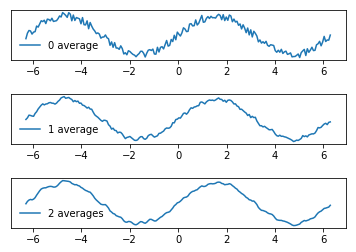### savefig function

To save the fig, we use the function savefig
For example:

savefig('test.pdf')  # save to pdf
savefig('test.svg')  # save to svg
savefig('test.pdf', transparent = true)  # make the background transparent
savefig('test.pdf', bbox_inches = 'tight') # reduce the surrounding space by setting the bounding box tight


## Meshgrid and contour

To generate a grid on the rectangle $[a,b] \times [c,d]$, we use the meshgrid command:

n = ... # number of discretization points along the x-axis
m = ... # number of discretization points along the x-axis
X,Y = meshgrid(linspace(a,b,n), linspace(c,d,m))


A rectangle discretized by meshgrid can be used to visualize the behavior of an iteration.
But first we use it to plot level curves of a function. This is done by the command contour.

We choose Rosenbrock’s banana function as an example:
$f(x,y) = (1-x)^2 + 100(y-x^2)^2$

rosenbrockfunction = lambda x,y: (1-x)**2 + 100*(y-x**2)**2
X,Y = meshgrid(linspace(-.5, 2., 100), linspace(-1.5, 4., 100))
Z = rosenbrockfunction(X, Y)
contour(X, Y, Z, logspace(-0.5,  3.5, 20, base = 10), cmap = 'gray')
title('Rosenbrock Function: $f(x,y) = (1-x)^2+100(y-x^2)^2$')
xlabel('x')
ylabel('y')

Text(0,0.5,'y')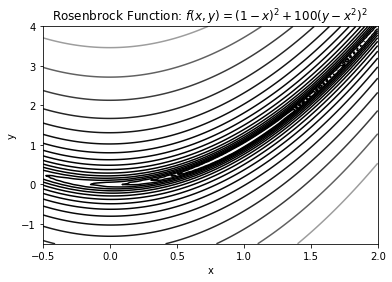the command contourf has the same use of contour but fills the plot with different colors according to different levels. Here we simply change the contour to contourf in the above code to see the output:

rosenbrockfunction = lambda x,y: (1-x)**2 + 100*(y-x**2)**2
X,Y = meshgrid(linspace(-.5, 2., 100), linspace(-1.5, 4., 100))
Z = rosenbrockfunction(X, Y)
contourf(X, Y, Z, logspace(-0.5,  3.5, 20, base = 10), cmap = 'gray')
title('Rosenbrock Function: $f(x,y) = (1-x)^2+100(y-x^2)^2$')
xlabel('x')
ylabel('y')

Text(0,0.5,'y')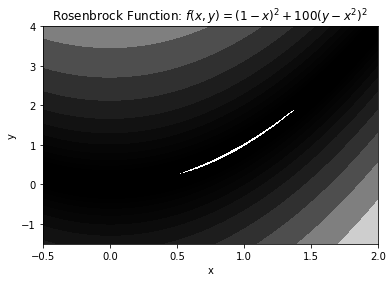And we continue with the above example of Rosenbrock Function.
We use Powell’s method to find the minimum of Rosenbrock Function.

import scipy.optimize as so

rosenbrockfunction = lambda x,y: (1-x)**2+100*(y-x**2)**2
X,Y=meshgrid(linspace(-.5,2.,100),linspace(-1.5,4.,100))
Z=rosenbrockfunction(X,Y)
cs=contour(X,Y,Z,logspace(0,3.5,7,base=10),cmap='gray')

rosen=lambda x: rosenbrockfunction(x,x)
solution, iterates = so.fmin_powell(rosen,x0=array([0,-0.7]),retall=True)
x,y=zip(*iterates)
plot(x,y,'ko') # plot black bullets
plot(x,y,'k:',linewidth=1) # plot black dotted lines
title("Steps of Powell's method to compute a minimum")
clabel(cs)

Optimization terminated successfully.
Current function value: 0.000000
Iterations: 16
Function evaluations: 462

<a list of 11 text.Text objects>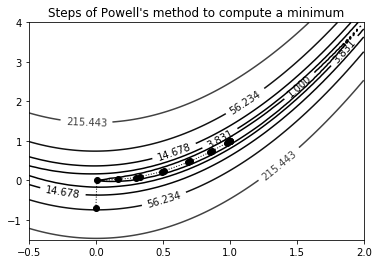The iterative method fmin_powell applies Powell’s method to find a minimum.

It is started by a given start value of $x_0$ and reports all iterates when the option retall=True is
given.

After sixteen iterations, the solution $x=0, y=0$ was found. The iterations are depicted
as bullets in the above contour plot.

PS:

1. to know about the function zip, you can check here.
2. contour also creates a contour set object that we assigned to the variable cs, which is then used by the command clabel to annotate the levels of the corresponding function values.

## images and contours

The following function will create a matrix of color values for the Mandelbrot fractal.

Consider a fixed point iteration, that depends on a complex parameter $c$:

$z_{n+1} = z_n^2 + c, with \space c \in \mathbb{C}$

Whether the sequence is bounded depends on the parameter $c$.

Here we consider the sequence to be bounded if the value in below the bound within maxit times iteration.

def mandelbrot(h,w, maxit=20):
X,Y = meshgrid(linspace(-2, 0.8, w), linspace(-1.4, 1.4, h))
c = X + Y*1j
z = c
exceeds = zeros(z.shape, dtype=bool)

for iteration in range(maxit):
z = z**2 + c
exceeded = abs(z) > 4
exceeds_now = exceeded & (logical_not(exceeds))
exceeds[exceeds_now] = True
z[exceeded] = 2 # limit the values to avoid overflow

return exceeds

imshow(mandelbrot(400,400),cmap='gray')
axis('off')

(-0.5, 399.5, 399.5, -0.5)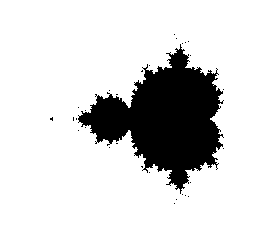The command imshow displays the matrix as an image. The selected color map shows the regions where the sequence appeared unbounded in white and others in black.

Here we used axis('off') to turn off the axis as this might be not so useful for images.

This blog is under a CC BY-NC-SA 3.0 Unported License